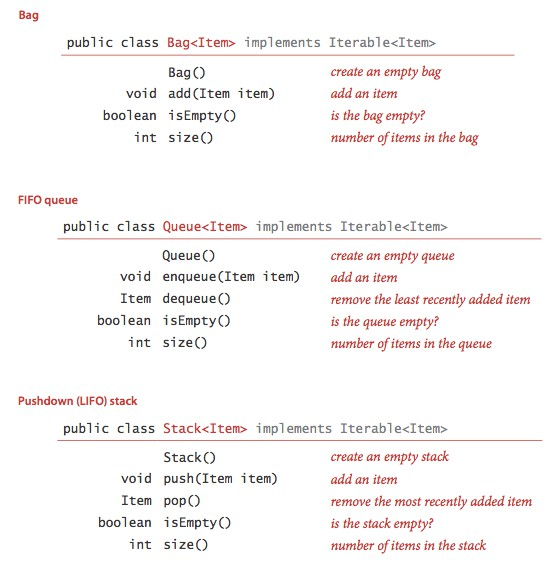﻿ C#数据类型实现背包、队列和栈_C#教程_脚本之家

# C#数据类型实现背包、队列和栈

更新时间：2022年04月15日 11:06:09   作者：Ruby_Lu

## 基础编程模型和数据抽象

• 1. 递归总有一个最简单的情况 —— 方法的第一条语句总是包含 return 的条件语句。
• 2. 递归调用总是尝试解决一个规模更小的子问题，这样递归才能收敛到最简单的情况。
• 3. 递归调用的父问题和尝试解决的子问题之间不应该有交集。

## 1. APIStack 和 Quene 都含有一个能够删除集合中特定元素的方法。

### 3. 下压栈

• 1. 将操作数压入操作数栈；
• 2. 将运算符压入运算符栈；
• 3. 忽略做括号；
• 4. 在遇到右括号时，弹出一个运算符，弹出所需数量的操作数，并将运算符和操作数的运算结果压入操作数栈。

## 2.用数组实现

```//想要数据类型可迭代，需要实现IEnumerable
public class ResizingStack<Item> : IEnumerable<Item>
{
private Item[] a = new Item;
private int N = 0;
public bool IsEmpty{ get {
return N == 0;
} }
public int Size { get {
return N;
} }
public int Count { get; set; }

/// <summary>
/// 使数组处于半满
/// </summary>
/// <param name="max"></param>
private void Resize(int max)
{
Count = 0;
Item[] temp = new Item[max];
for(var i = 0;i<N;i++)
{
temp[i] = a[i];
Count++;
}
a = temp;
}

public void push(Item item)
{
if (N == a.Length)
Resize(a.Length * 2);
a[N++] = item;
}

public Item Pop()
{
Item item = a[--N];
a[N] = default(Item); //避免对象游离
if (N > 0 && N == a.Length / 4)
Resize(a.Length/2);
return item;
}

IEnumerator<Item> IEnumerable<Item>.GetEnumerator()
{
return new ResizingStackEnumerator<Item>(a);
}

public IEnumerator GetEnumerator()
{
return new ResizingStackEnumerator<Item>(a);
}

}
class ResizingStackEnumerator<Item> : IEnumerator<Item>
{
private Item[] a;
private int N = 0;
public ResizingStackEnumerator(Item[] _a)
{
a = _a;
N = a.Length-1;
}

public object Current => a[N--];

Item IEnumerator<Item>.Current => a[N--];

public void Dispose()
{
throw new NotImplementedException();
}

public bool MoveNext()
{
return N > 0;
}

public void Reset()
{
throw new NotImplementedException();
}
}```

## 3.链表

```class Node<Item>
{
public Item item { get; set; }
public Node<Item> Next { get; set; }
}```

### 1.构造链表### 2.在表头插入结点### 3.从表头删除结点### 4.在表尾插入结点### 5.其他位置的插入和删除操作

• 1. 删除指定的结点
• 2. 在指定结点前插入一个新结点

### 6. 遍历

```public class Bag<Item>
{
private Node<Item> first;
{
Node<Item> oldFirst = first;
first = new Node<Item>() {
item = item,
Next = oldFirst
};

}
}```
```Bag<int> bags = new Bag<int>();
for (var i = 0; i < 10; i++)
{
}

for (var x = bags.first; x != null; x = x.Next)
{
Console.WriteLine(x.item);
}```

```public class Bag<Item>: IEnumerable<Item>
{
public Node<Item> first;
{
Node<Item> oldFirst = first;
first = new Node<Item>() {
item = item,
Next = oldFirst
};

}

public IEnumerator<Item> GetEnumerator()
{
return new LineEnumerator<Item>(first);
}

IEnumerator IEnumerable.GetEnumerator()
{
return new LineEnumerator<Item>(first);
}
}

public class LineEnumerator<Item> : IEnumerator<Item>
{
public Node<Item> first;
public LineEnumerator(Node<Item> _first)
{
first = _first;
}
public Item Current { get {
var oldfirst = first;
first = first.Next;
return oldfirst.item;
} }

object IEnumerator.Current => first;

public void Dispose()
{
return;
}

public bool MoveNext()
{
if (first != null)
return true;
return false;
}

public void Reset()
{
throw new NotImplementedException();
}
}```
```public static void LineTest()
{
Bag<int> bags = new Bag<int>();
for (var i = 0; i < 10; i++)
{
}

foreach(var bag in bags)
{
Console.WriteLine(bag);
}
}```

## 5. 用链表实现栈

Stack API 中 Pop() 删除一个元素，按照前面的从表头删除结点实现，Push() 添加一个元素，按照前面在表头插入结点。

```public class Stack<Item> : IEnumerable<Item>
{
public Node<Item> first;
private int N;

public bool IsEmpty()
{
return first == null; //或 N == 0
}

public int Size()
{
return N;
}

public void Push(Item item)
{
Node<Item> oldfirst = first;
first = new Node<Item>() {
item = item,
Next = oldfirst
};
N++;
}

public Item Pop()
{
Item item = first.item;
first = first.Next;
N--;
return item;
}

public IEnumerator<Item> GetEnumerator()
{
return new StackLineIEnumerator<Item>(first);
}

IEnumerator IEnumerable.GetEnumerator()
{
return new StackLineIEnumerator<Item>(first);
}
}

public class StackLineIEnumerator<Item> : IEnumerator<Item>
{
private Node<Item> first;
public StackLineIEnumerator(Node<Item> _first)
{
first = _first;
}
public Item Current { get {
var oldfirst = first;
first = first.Next;
return oldfirst.item;
} }

object IEnumerator.Current => throw new NotImplementedException();

public void Dispose()
{
return;
}

public bool MoveNext()
{
return first != null;
}

public void Reset()
{
throw new NotImplementedException();
}
}```

• 1. 可以处理任意类型的数据；
• 2. 所需的空间总是和集合的大小成正比；
• 3. 操作所需的时间总是和集合的大小无关；

## 6. 用链表实现队列

```    public class Quene<Item> : IEnumerable<Item>
{
public Node<Item> first;
public Node<Item> last;
private int N;

public bool IsEmpty()
{
return first == null;
}

public int Size()
{
return N;
}

public void Enquene(Item item)
{
var oldlast = last;
last = new Node<Item>() {
item = item,
Next = null
};

if (IsEmpty())
first = last;
else
oldlast.Next = last;
N++;
}

public Item Dequene()
{
if (IsEmpty())
throw new Exception();
Item item = first.item;
first = first.Next;
if (IsEmpty())
last = null;
N--;
return item;
}

public IEnumerator<Item> GetEnumerator()
{
return new QueneLineEnumerator<Item>(first);
}

IEnumerator IEnumerable.GetEnumerator()
{
return new QueneLineEnumerator<Item>(first);
}
}
public class QueneLineEnumerator<Item> : IEnumerator<Item>
{
private Node<Item> first;
public QueneLineEnumerator(Node<Item> _first)
{
first = _first;
}
public Item Current { get {
var oldfirst = first;
first = first.Next;
return oldfirst.item;
} }

object IEnumerator.Current => throw new NotImplementedException();

public void Dispose()
{
return;
}

public bool MoveNext()
{
return first != null ;
}

public void Reset()
{
throw new NotImplementedException();
}
}```

## 7. 总结

• 1. 定义 API
• 2. 根据特定的应用场景开发用例代码
• 3. 描述一种数据结构（即一组值的表示），并在 API 的实现中根据它定义类的实例变量。
• 4. 描述算法，即实现 API，并根据它应用于用例
• 5. 分析算法的性能

到此这篇关于C#数据类型实现背包、队列和栈的文章就介绍到这了。希望对大家的学习有所帮助，也希望大家多多支持脚本之家。